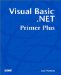# Review Questions

1:

What is an array?

A1:

An array is a data structure that allows you to reference multiple values of a data type by using a single name .

2:

What's wrong with the following data declaration?

` Dim MyArray as Integer `
A2:

First, the statement is attempting to define a data item, not to declare it. The problem is that Visual Basic .NET does not see the definition as an array definition. Instead, it defines a single integer variable named MyArray . To make an array of 10 integers, you would define it like this:

` Dim MyArray(9) as Integer `
3:

In the following statement, how much storage is required for the array?

` Dim MyArray(10) as Double `
A3:

Visual Basic .NET uses the number 10 in this statement to determine how much storage to request from the operating system. Each Double data type takes 8 bytes. Remember that arrays start with element 0 by default, and the number 10 in the statement is the highest subscript allowed for the array. Therefore, elements 0 through 10 are valid, which means 11 numbers have 8 bytes each ”88 bytes total. Therefore, Windows returns 88 bytes of storage.

4:

What role does the type specifier play in fetching an array value?

A4:

Suppose you have an array named MyArray that contains 10 integers that are stored starting at memory location 1000 . Because the type specifier tells you the type for the data item being stored, and because each integer takes 4 bytes of storage, the array looks like this in memory:

 1004 1012 1020 1028 1036 1 2 3 4 5 6 7 8 9 1000 1008 1016 1024 1032

To read element 5 (which is actually subscript 4), Visual Basic .NET takes the lvalue of 1000 , and adds the product of the subscript ( 4 ) multiplied by the storage required for each element of the array (2 bytes), and adds that product to the lvalue . This gives Visual Basic .NET the proper memory address to fetch the value. Using the information provided:

 MemoryAddress = lvalue + ( Subscript x BytesPerItem ) MemoryAddress = 1000 + (4 x 4) MemoryAddress = 1016

Therefore, MyArray(4) is located at memory address 1016 , which agrees with the array diagram. It also follows that Visual Basic .NET uses the type specifier to figure out the proper offset from the lvalue to fetch the proper element of the array.

5:

How do you define a dynamic array?

A5:

Defining a dynamic array is a two-step process. First, you define a reference to the array with this statement:

` Dim MyArray() as String `

This places a reference variable in the symbol table, but no actual array storage is yet defined. At some later point in the program, you need this statement:

` ReDim MyArray(9) `

which defines an array of 10 strings (that is, 0 “9). If you later want to increase the array so that it has more than 10 elements, you would use this:

` ReDim Preserve MyArray(19) `

This causes 10 new (empty) strings to be added to the array, but it preserves the string content for the first 10 elements.

6:

Suppose you want to define an array whose first element is 1 instead of 0. How would you define the array?

A6:

7:

How do you define a multidimensional array that will be used to store a table of values? The first dimension should be used to hold the number, and the second dimension should hold the square of that number. You should make the array large enough to hold the values 0 through 100.

A7:

This is the statement to define this multidimensional array:

` Dim MyArray(100,1) as Integer `

Too often, students define this array as MyArray(100,100) , which is wasteful . The proper assignment for the number 5, for example, would be this:

` MyArray(5,0) = 5  MyArray(5,1) = 25 `
8:

Suppose you have a text box named txtNumber into which the user types a number. Further assume that an array named MyArray() is capable of storing 1,000 numbers. The value typed into txtNumber is to be copied into the element that matches the number that was typed in. For example, if the text box holds the value 100, MyArray(100) should equal 100 .

A8:

The following statements should do the trick:

` Dim MyArray(1000) As Integer, Index As integer  Index = cint(txtNumber.Text) MyArray(Index) = Index `Visual Basic .NET Primer Plus
ISBN: 0672324857
EAN: 2147483647
Year: 2003
Pages: 238
Authors: Jack Purdum

Similar book on Amazon